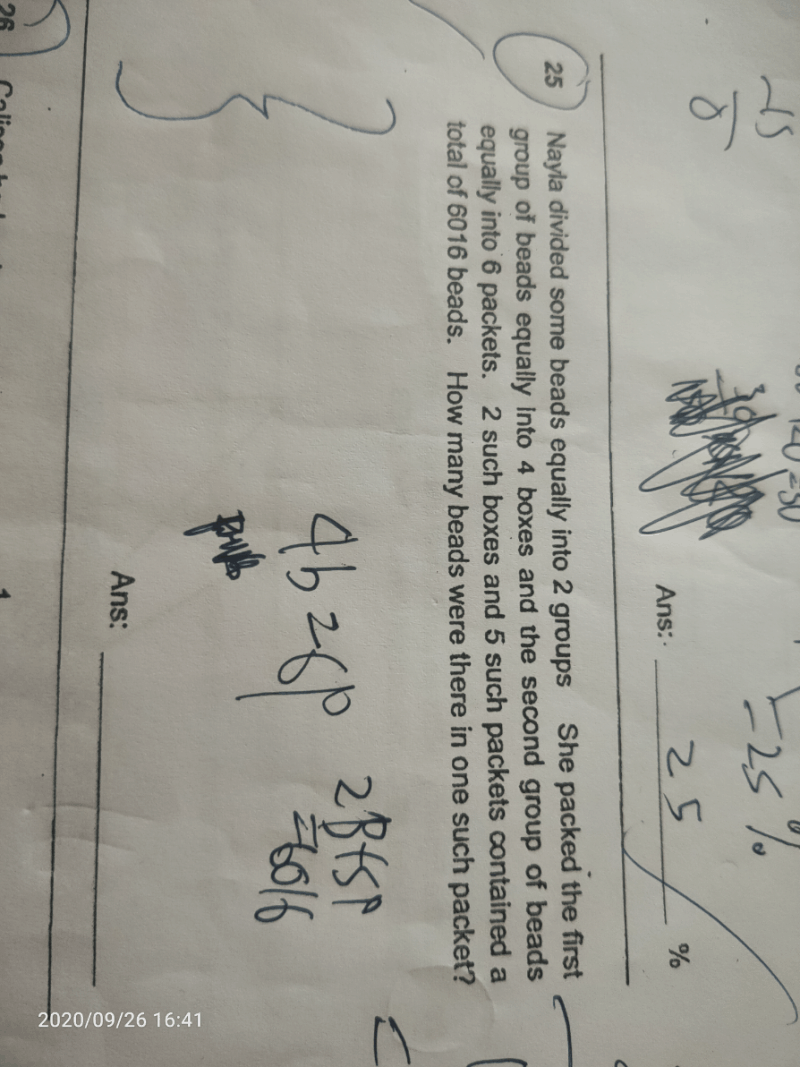# QuestionSolve using Simultaneous Equations.

Let boxes be B and packets be P,

1st equation –> 4B – 6P = 0

2nd equation –> 2B + 5P = 6016

Make Bs the same,

1st equation –> 4B – 6P = 0

2nd equation*2 –> 4B + 10P = 12 032

When 4B is cancelled off, you get

16P = 12 032

P = 12 032 ÷ 16 = 752

Hope you understand and good luck for your PSLE!